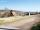# Annulus

The radius of the larger circle is 8cm, the radius of smaller is 5cm. Calculate the contents of the annulus.

Result

S =  122.522 cm2

#### Solution:Leave us a comment of example and its solution (i.e. if it is still somewhat unclear...):Be the first to comment!## Next similar examples:

1. 22/7 circleCalculate approximately area of a circle with radius 20 cm. When calculating π use 22/7.
2. Circle - easy 2The circle has a radius 6 cm. Calculate:
3. Two circlesTwo circles with a radius 4 cm and 3 cm have a center distance 0.5cm. How many common points have these circles?
4. Bicycle wheelAfter driving 157 m bicycle wheel rotates 100 times. What is the radius of the wheel in cm?
5. CableCable consists of 8 strands, each strand consists of 12 wires with diameter d = 0.5 mm. Calculate the cross-section of the cable.
6. Diameter to areaFind the area of a circle whose diameter is 26cm.
7. SidewalkThe city park is a circular bed of flowers with a diameter of 8 meters, around it the whole length is 1 meter wide sidewalk . What is the sidewalk area?
8. Circle - simpleThe circumference of a circle is 930 mm. How long in mm is its diameter?
9. Clock handsThe second hand has a length of 1.5 cm. How long does the endpoint of this hand travel in one day?
10. AcreagePlot has a diamond shape, its side is 25.6 m long and the distance of the opposite sides is 22.2 meters. Calculate its acreage.
11. HotelThe hotel has a p floors each floor has i rooms from which the third are single and the others are double. Represents the number of beds in hotel.
12. Round it0.728 round to units, tenths, hundredths.
13. Simple equationSolve the following simple equation: 2. (4x + 3) = 2-5. (1-x)
14. Cupcakes 2Susi has 25 cupcakes. She gives 4/5. How much does she have left?
15. Write 2Write 791 thousandths as fraction in expanded form.
16. WithdrawalIf I withdrew 2/5 of my total savings and spent 7/10 of that amount. What fraction do I have in left in my savings?
17. TeacherTeacher Rem bought 360 pieces of cupcakes for the outreach program of their school. 5/9 of the cupcakes were chocolate flavor and 1/4 wete pandan flavor and the rest were vanilla flavor. How much more chocolate flavor cupcakes than vanilla flavor?